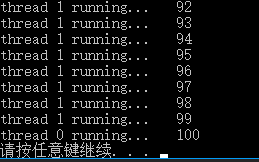﻿ C++多线程之互斥锁与死锁_C 语言_脚本之家
C 语言# C++多线程之互斥锁与死锁

## 1.前言

```class msgList
{
private:
list<int>mylist;   //用list模仿一个消息队列

public:
void WriteList()   //向消息队列中写入消息(以i作为消息)
{
for (int i = 0; i<100000; i++)
{
cout << "Write : " << i <<endl;
mylist.push_back(i);
}
return;
}
{
for(int i=0;i<100000;i++)
{
if (!mylist.empty())
{
cout << "Read : " << mylist.front() << endl;
mylist.pop_front();
}
else
{
cout << "Message List is empty!" << endl;
}
}
}
};
int main()
{
msgList mlist;
//等待线程结束
pwrite.join();

return 0;
}```## 2.互斥锁

### 2.1 互斥锁的特点

1. 原子性：把一个互斥量锁定为一个原子操作，这意味着如果一个线程锁定了一个互斥量，没有其他线程在同一时间可以成功锁定这个互斥量；

2. 唯一性：如果一个线程锁定了一个互斥量，在它解除锁定之前，没有其他线程可以锁定这个互斥量；

3. 非繁忙等待：如果一个线程已经锁定了一个互斥量，第二个线程又试图去锁定这个互斥量，则第二个线程将被挂起（不占用任何cpu资源），直到第一个线程解除对这个互斥量的锁定为止，第二个线程则被唤醒并继续执行，同时锁定这个互斥量。

### 2.2 互斥锁的使用

```class msgList
{
private:
list<int>mylist;   //用list模仿一个消息队列
mutex mtx;   //创建互斥锁对象
public:
void WriteList()   //向消息队列中写入消息(以i作为消息)
{
mtx.lock();
for (int i = 0; i<100000; i++)
{
cout << "Write : " << i <<endl;
mylist.push_back(i);
}
mtx.unlock();
return;
}
//.......
};```

```class msgList
{
private:
list<int>mylist;   //用list模仿一个消息队列
mutex mtx;   //创建互斥锁对象
public:
void WriteList()   //向消息队列中写入消息(以i作为消息)
{
for (int i = 0; i<100000; i++)
{
mtx.lock();
cout << "Write : " << i <<endl;
mylist.push_back(i);
mtx.unlock();
}
return;
}
//.......
};```

```class msgList
{
private:
list<int>mylist;
mutex m;
int i = 0;
public:
void WriteList()
{
while(i<1000)
{
mylist.push_back(i++);
}
return;
}
void showList()
{
for (auto p = mylist.begin(); p != mylist.end(); p++)
{
cout << (*p) << " ";
}
cout << endl;
cout << "size of list : " << mylist.size() << endl;
return;
}
};
int main()
{
msgList mlist;

pwrite0.join();
pwrite1.join();
cout << "threads end!" << endl;

mlist.showList();  //子线程结束后主线程打印list
return 0;
}``````class msgList
{
private:
list<int>mylist;
mutex m;
int i = 0;
public:
void WriteList()
{
while(i<1000)
{
m.lock();//加锁
mylist.push_back(i++);
m.unlock(); //解锁
}
return;
}
// ......
};``````void WriteList()
{
while(i<10000)
{
m.lock();
if (i >= 10000)
{
m.unlock();   //退出之前必须先解锁
break;
}
mylist.push_back(i++);
m.unlock();
}
return;
}```### 2.3 std::lock_guard

```void WriteList()
{
while(i<10000)
{
lock_guard<mutex> guard(m);  //创建lock_guard的类对象guard，用互斥量m来构造
//m.lock();
if (i >= 10000)
{
//m.unlock();   //由于有了guard，这里就无需unlock()了
break;
}
mylist.push_back(i++);
//m.unlock();
}
return;
}```

## 3.死锁

### 3.2 死锁的例子

```mutex m0,m1;
int i = 0;
void fun0()
{
while (i < 100)
{
lock_guard<mutex> g0(m0);  //线程0加锁0
lock_guard<mutex> g1(m1);  //线程0加锁1
cout << "thread 0 running..." << endl;
}
return;
}
void fun1()
{
while (i < 100)
{
lock_guard<mutex> g1(m1);  //线程1加锁1
lock_guard<mutex> g0(m0);  //线程1加锁0
cout << "thread 1 running...   "<< i << endl;
}
return;
}
int main()
{
p0.join();
p1.join();
return 0;
}```### 3.3 死锁的解决方法

①按顺序加锁

```mutex m0,m1;
int i = 0;
void fun0()
{
while (i < 100)
{
lock_guard<mutex> g0(m0);  //线程0加锁0
lock_guard<mutex> g1(m1);  //线程0加锁1
cout << "thread 0 running..." << endl;
}
return;
}
void fun1()
{
while (i < 100)
{
lock_guard<mutex> g0(m0);  //线程1加锁0
lock_guard<mutex> g1(m1);  //线程1加锁1
cout << "thread 1 running...   "<< i << endl;
}
return;
}
int main()
{
p0.join();
p1.join();
return 0;
}```②同时上锁

```mutex m0,m1;
int i = 0;
void fun0()
{
while (i < 100)
{
lock(m0,m1);
cout << "thread 0 running..." << endl;
}
return;
}
void fun1()
{
while (i < 100)
{
lock(m0,m1);
cout << "thread 1 running...   "<< i << endl;
}
return;
}
int main()
{